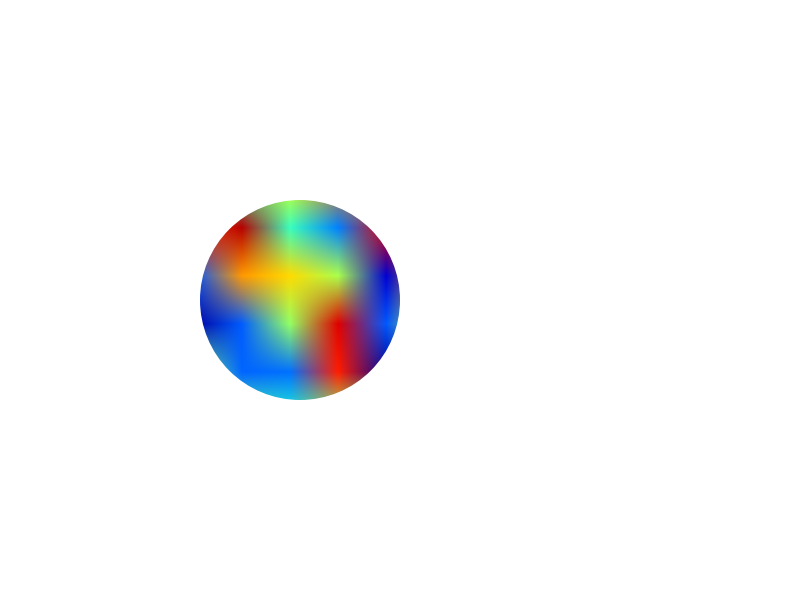# Dog Ear?

Hello,

I am relatively new to matplotlib but I get the basics. What I need to do though is a slightly customized graph area. There are two plots on the same graph - a parabola and a straight line (with a negative slope) north east of the parabola. This graph has grid lines. Simple enough, but now I want to remove the graph area to the right of the line. Think of a dog-eared page in a book. Basically that top right triangle is gone.

Can I do this in MPL or do I need to do some post processing in PIL? Much thanks for any help.

-Andrew

Hi Andrew,

if I understand correctly "set_clip_path" is what you want.
The usage was discussed in the following threads, where John Hunter also
proposed the example code added below.

The only example I found on the web and in the svn-examples about clip_path is
http://matplotlib.sourceforge.net/examples/pylab_examples/image_clip_path.html?highlight=clip_path

For developers: Could it be useful to include John's example in the online
screenshots / gallery or did I miss it?

best regards Matthias

"""
Clipping to arbitrary patches and paths
"""
import numpy as np
import matplotlib.pyplot as plt
import matplotlib.path as path
import matplotlib.patches as patches

fig = plt.figure()
ax = fig.add_subplot(111, frameon=False, xticks=[], yticks=[])

im = ax.imshow(np.random.rand(10,10))

im.set_clip_path(patch)

plt.show()···

On Wednesday 15 April 2009 09:01:56 Andrew Kelly wrote:

Hello,I am relatively new to matplotlib but I get the basics. What I need
to do though is a slightly customized graph area. There are two plots on
the same graph - a parabola and a straight line (with a negative slope)
north east of the parabola. This graph has grid lines. Simple enough, but
now I want to remove the graph area to the right of the line. Think of a
dog-eared page in a book. Basically that top right triangle is gone.

Can I do this in MPL or do I need to do some post processing in PIL? Much
thanks for any help.

-Andrew